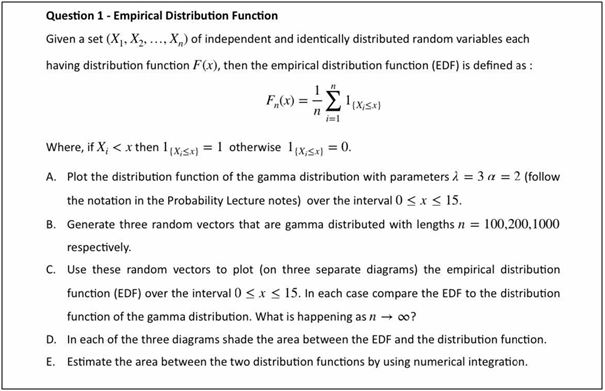# Empirical Distribution and Taylor’s Expansion Assignment Help

Do you want to submit impressive solutions for your assignment? Take our empirical distribution and Taylor’s expansion assignment help. We are associated with skillful MATLAB homework tutors who are acquainted with these complicated topics. They will not only help you prepare correct MATLAB scripts but also ensure that you do not miss your deadline. You should opt for our empirical distribution and Taylor’s expansion homework help if you are tired of securing dismal grades.

## Empirical Distribution Function## MATLAB Script

clc, clear all, close all

%% Question 1

%% part A)

lambda = 3;

alpha = 2;

xspan = linspace(0, 15, 1000); % 1000 points between 0 and 15

n = length(xspan);

% Generate data X

X = rand(1,n)*15;

% Generate F_n (x)

F = zeros(n);

for xi = xspan

fori = 1:n

if X(i) <= xi

l = 1;

else

l = 0;

end

F(i) = F(i) + l;

end

end

F = 1/n *F;

% Calculate gamma distrbution

f = zeros(n,1);

fori = 1:n

xi = xspan(i);

if xi > 0

f(i) = (lambda^alpha)*xi^(alpha-1) *exp(-lambda*xi)/gamma(alpha);

else

f(i) = 0;

end

end

figure

plot(xspan, f), grid on

xlabel('X');

ylabel('Value');

title('Gamma Distribution');

%% part B)

x1 = rand(1,100);

x2 = rand(1,200);

x3 = rand(1,1000);

%% Part C)

% Empirical distributions

F = zeros(n);

fori = 1:n

Xi = X(i);

if Xi

F = F + 1;

end

end

% Generate F_n (x)

F1 = zeros(100,1);

xspan1 = linspace(0, 15, 100);

for xi = xspan1

fori = 1:100

if x1(i) <= xi

l = 1;

else

l = 0;

end

F1(i) = F1(i) + l;

end

end

F1 = 1/100 *F1;

% Calculate gamma distrbution

f1 = zeros(100,1);

fori = 1:100

xi = xspan1(i);

if xi > 0

f1(i) = (lambda^alpha)*xi^(alpha-1) *exp(-lambda*xi)/gamma(alpha);

else

f1(i) = 0;

end

end

F2 = zeros(200,1);

xspan2 = linspace(0, 15, 200);

for xi = xspan2

fori = 1:200

if x2(i) <= xi

l = 1;

else

l = 0;

end

F2(i) = F2(i) + l;

end

end

F2 = 1/200 *F2;

% Calculate gamma distrbution

f2 = zeros(200,1);

fori = 1:200

xi = xspan2(i);

if xi > 0

f2(i) = (lambda^alpha)*xi^(alpha-1) *exp(-lambda*xi)/gamma(alpha);

else

f2(i) = 0;

end

end

F3 = zeros(1000,1);

xspan3 = linspace(0, 15, 1000);

for xi = xspan3

fori = 1:1000

if x3(i) <= xi

l = 1;

else

l = 0;

end

F3(i) = F3(i) + l;

end

end

F3 = 1/1000 *F3;

% Calculate gamma distrbution

f3 = zeros(1000,1);

fori = 1:1000

xi = xspan3(i);

if xi > 0

f3(i) = (lambda^alpha)*xi^(alpha-1) *exp(-lambda*xi)/gamma(alpha);

else

f3(i) = 0;

end

end

% Plot

figure

subplot(3,1,1)

plot(xspan1, F1), grid on

hold on

plot(xspan1, f1)

title('n = 100')

subplot(3,1,2)

plot(xspan2, F2), grid on

hold on

plot(xspan2, f2)

title('n = 200')

subplot(3,1,3)

plot(xspan3, F3), grid on

hold on

plot(xspan3, f3)

title('n = 1000')

%%%%%%%%%%%%%%%%%%%%%%%%%%%%%%%%%%%%%%%%%

%% It can be seen that, as n increases, the "dispersion" between the points decreases, and the curve becomes a straight line with a negative slope

%%%%%%%%%%%%%%%%%%%%%%%%%%%%%%%%%%%%%%%%%

%% Part D)

xs1 = [xspan1, fliplr(xspan1)];

xs2 = [xspan2, fliplr(xspan2)];

xs3 = [xspan3, fliplr(xspan3)];

subplot(3,1,1)

subplot(3,1,2)

subplot(3,1,3)

%% Part E)

fprintf("The area between the shaded region for n = 100 is: %.4f\n", trapz(xs1, shaded1));

fprintf("The area between the shaded region for n = 200 is: %.4f\n", trapz(xs2, shaded2));

fprintf("The area between the shaded region for n = 1000 is: %.4f\n", trapz(xs3, shaded3));

##### Question 2 Solution

clc, clear all, close all

syms x y

f(x,y) = exp(-x^2 - y^2);

%% Part 1: Function in TaylorExpansion.m

T(x,y) = TaylorExpansion(f, 3);

%% part 2

for n = 0:3

fprintf("The Taylor Expansion for %d terms is:\n", n);

T(x,y) = TaylorExpansion(f, n)

end

%% Part 3

xspan = linspace(-2, 2, 50);

yspan = linspace(-2, 2, 50);

figure

hold on

legends = {};

for n = 0:6

T(x,y) = TaylorExpansion(f, n);

plot3(xspan, yspan, double(T(xspan, yspan)));

legends{n+1} = sprintf("%d terms\n", n);

end

legend(legends);

xlabel('X');

ylabel('Y');

zlabel('Z');

grid minor

view(3)

%% In the first terms, the function looks like a straight line on the X-Y plane. However, as n increases. the function

% becomes a polynomial that depends of both x and y. and it has the shape

% of a W. As n increases, the value of the curve decreases, but it

% maintains its shape.

##### Taylor’s SeriesExpansion Function

function T = TaylorExpansion(f, n)

syms x y

a = 0;

b = 0;

T(x,y) = sym(0);

forni = 0:n-1

fornj = 0:n-1

T(x,y) = T(x,y) + ((x-a)^ni)*((y-b)^(nj))/(factorial(ni)*factorial(nj)) *subs(diff(diff(f,y,nj),x,ni), [x,y], [0,0]);

end

end

end